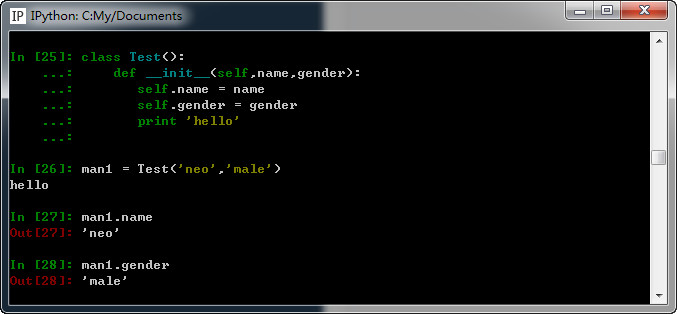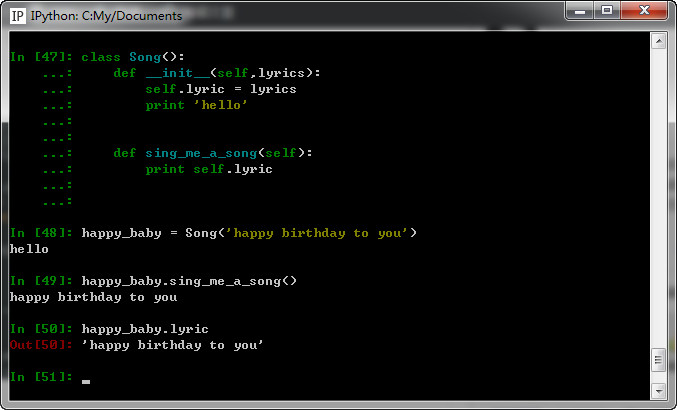# Python __init__ 用法

XAMPP教程 1453浏览

 `1` `class` `Rectangle():`
 `2` `    ``def getPeri(self,a,b):`
 `3` `        ``return` `(a + b)*2`
 `4` `    ``def getArea(self,a,b):`
 `5` `        ``return` `a*b`
 `6`
 `7` `rect = Rectangle()`
 `8` `print``(rect.getPeri(3,4))`
 `9` `print``(rect.getArea(3,4))`
 `10` `print``(rect.__dict__)`

 `1` `14`
 `2` `12`
 `3` `{}`

 `1` `class` `Rectangle():`
 `2` `    ``def __init__(self,a,b):`
 `3` `        ``self.a = a`
 `4` `        ``self.b = b`
 `5` `    ``def getPeri(self):`
 `6` `        ``return` `(self.a + self.b)*2`
 `7` `    ``def getArea(self):`
 `8` `        ``return` `self.a * self.b`
 `9`
 `10` `rect = Rectangle(3,4)`
 `11` `print``(rect.getPeri())`
 `12` `print``(rect.getArea())`
 `13` `print``(rect.__dict__)`

 `1` `14`
 `2` `12`
 `3` `{``'a'``: 3, ``'b'``: 4}`

__init__ 的意思是：initialize，初始化，因此可以理解实例化出某个类的对象时调用的初始化方法。

1、它位于类内部，可以让你在里面先做一些初始化工作，例如定义一些变量供后面的其它方法使用。

 `1` `class` `Song():`
 `2` `    ``def __init__(self,lyrics):`
 `3` `        ``self.lyric = lyrics`
 `4`
 `5`
 `6` `    ``def sing_me_a_song(self):`
 `7` `        ``for` `line in self.lyric:`
 `8` `            ``print` `line`
 `9`
 `10` `happy_baby = Song([``'happy birthday to you'``,`
 `11` `                   ``'I do not want to get sued'``,`
 `12` `                   ``'So i will stop right there'``])`
 `13`
 `14` `bulls_on_parade = Song([``'They rally around the family'``,`
 `15` `                        ``'with pockets full of shells'``])`
 `16`
 `17` `happy_baby.sing_me_a_song()`
 `18` `bulls_on_parade.sing_me_a_song()`

2、定义类的时候，若是添加__init__方法，那么在创建类的实例的时候，实例会自动调用这个方法，一般用来对实例的属性进行初使化。比如：